# RBSE Class 9 Science Chapter 9: Force and Motion Important Textbook Questions and Solutions

In this chapter, we will study about force and motion. Before proceeding with the study of force and motion, we will learn as to how various physical quantities are expressed. Generally, the physical quantities are expressed by their unit and numeric value. While expressing some physical quantities, even the direction is to be mentioned along with the unit and numeric value. Physical quantities are classified into Scalar quantities, Vector quantities.

These solutions of Class 9 Science Chapter 9 are designed by our subject experts to facilitate smooth and precise understanding of concepts. These RBSE Class 9 solutions of Chapter 9: Force and Motion have detailed step-by-step explanations of problems given in the textbooks. It covers all the questions mentioned in the textbook. Solving the questions will help in grasping the concepts correctly.

You can also get all subjects Class 9 solutions for Rajasthan Board here.

### RBSE Class 9 Science Chapter 9: Objective Textbook Questions and Solutions

Q1. Which of the following is a vector quantity:

(A) Work

(B) Time

(C) Mass

(D) Gravitational force

Q2. Two forces of 4N and 3N are working on the same body in opposite directions. Then the magnitude of the force on the particle will be:

(A) 5N

(B) 7N

(C) IN

(D) Between IN and 7N

Q3. Rate of change of velocity is:

(A) Force

(B) Momentum

(C) Acceleration

(D) Displacement

Q4. Unit of momentum is:

(A) Newton meter

(B) Newton Kilogram/meter

(C) Newton meter/second

(D) Kilogram meter/second

Q5. The velocity time graph of an object moving with uniform velocity is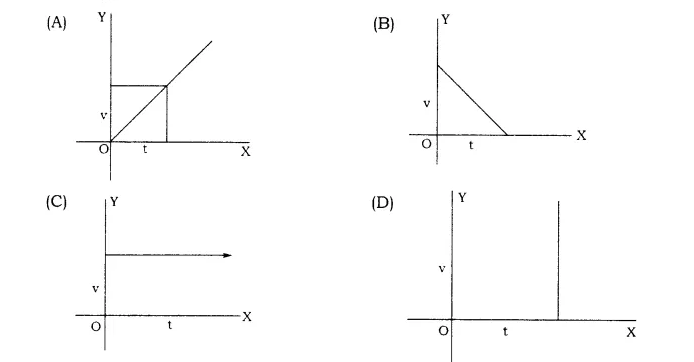Q6. The momentum of an object depends upon:

(A) Mass of the object

(B) Displacement of the object

(C) Time taken for displacement

(D) All of the above

Q7. The equation relating the Force (F) mass (m) and acceleration (a) is:

(a) F = ma

(b) m = aF

(c) a = mF

(d) ma =

Q8. Unit of force is:

(A) Kilogram – meter – second

(B) Kilogram – meter – second2

(C) Kilogram – meter / second2

(D) Kilogram – meter / second

Q9. If a body moves in a straight line with a uniform velocity and no external force is applied to it, then

(A) Its velocity will increase

(B) Velocity will remain constant

(C) The body will stop after some time

(D) Speed will increase

Q10. The inertia of an object depends upon:

(A) Center of gravity of the object

(B) Mass of the object

(C) Gravitational acceleration

(D) Shape of the object

Q11. An object of 5 Kg weight is moving in a simple line with an acceleration of 10m/s. The magnitude of the force working on the object will be:

(A) 50 N

(B) 0.5 N

(C) Zero

(D) 2 N

Q12. When a force is applied on an object:

(A) Its motion may change

(B) The direction of its motion may change

(C) Its shape may change

(D) All of the above

Q13. The weight of a body having mass 1 Kg will be:

(A) 1 Newton

(B) 9.08 Newton

(C) 9.8 Newton

(D) 8.9 Newton

Q14. If the mass of an object is m, velocity is v and acceleration is a, then its momentum p will be:

(A) p = ma

(B) p = mv

(C) p = m/v

(D) p = v/m

Q15. A body cannot change its state of rest or of motion because of its:

(A) mass

(B) weight

(C) acceleration

(D) inertia

Q16. If the force applied on the surface of an object is doubled, then the pressure will:

(A) reduce to half

(B) not change

(C) double itself

(D) become four times

### RBSE Class 9 Science Chapter 9: Very Short Answer Type Questions and Solutions

Q17. What will be the acceleration of a body moving with a uniform velocity of 40m/s after 10s?

Answer: When an object is moving in constant velocity then change in acceleration would be zero. i.e. u = v = 40m/s.

a = v – u/t

= 40 – 40/10

=0/10

=0m/s2

Q18. A moving body of mass m and having a velocity u collides with the wall and rebounds with the velocity u. What will be the change in its momentum?

Answer: The change in its momentum = mu – (- mu) = 2mu

Q19. What will be the distance covered in 30 mins by a train moving with a velocity of 20km/h.

Velocity = Speed / Time

Hence, Speed = Velocity x Time

Given, Velocity = 20 Km / Hour

Time = 30 Mins

Converting time from mins to hour = 0.5 Hour

Therefore, Speed = 0.5 x 20 = 10 Km/Hour

From Formula, we know

Distance = Speed x Time

Distance = 10 x 0.5 = 5 Kms

Q20. The area between the velocity time graph of a moving object and its time axis is equal to what?

Answer: The area between the velocity time graph of a moving object and its time axis is equal to the distance travelled in the given time interval.

Q21. The principle of rocket is based on which one of the Newton’s laws?

Answer: The principle of rocket is based on Newton’s third law of motion, the law of action and reaction.

Q22. What is the direction of the force of friction of a moving bicycle?

Answer: A frictional force is always applied opposite to the direction of motion. So, the frictional force acts in the direction opposite to the direction in which the cycle is moving, at the point of contact of wheels with the road.

Q23. Why does a cricket player pull his hands gradually with the moving ball while holding a catch?

Answer: A cricket player pulls his hands gradually with the moving ball while holding a catch to decrease the rate of change of its momentum by increasing the time.

Q24. Why does a player run for some distance prior to jumping in a high jump /broad jump event.

Answer: A player runs for some distance prior to jumping in a high jump /broad jump event to acquire the inertia of motion.

Q25. Why does a person standing in a moving bus fall in the forward direction, if the bus halts suddenly?

Answer: The difference in inertia of rest and inertia of motion causes this action.

Q26. What will be the magnitude of the force on a body moving with constant velocity?

Answer: The magnitude of the force on a body moving with constant velocity will be zero.

Q27. What efforts should be made by a person standing in the middle of a frozen lake to reach the shore?

Answer: The person should exert a push on the ice, taking help of a stick, backwards. Due to this, the person will be pushed forward and thus sliding on the surface of the ice, the person will reach the shore.

Q28. What is 1 Newton force?

Answer: 1 Newton force is that amount of force, which when it acts on a body of mass 1 kg produces an acceleration of 1/s2.

Q29. What is Inertia?

Answer: A body remains in its state of rest or in a state of motion along a simple line, until and unless an unbalanced force acts upon it. The tendency of a body to resist a change in the state of rest or of motion is known as its inertia. This is Newton’s First Law of Motion.

Q30. On applying brakes the vehicle stops. What will be the momentum of the vehicle in the process?

Answer: The major part of the momentum of the vehicle will go to earth and the remaining part is transferred to molecules.

Q31. What is the momentum of the gun and its bullet before it is fired?

Answer: The momentum of the gun and its bullet before it is fired will be zero.

Q32. What is Thrust?

Answer: The vertical force applied to the surface of an object is known as thrust. Its SI

unit is Newton.

Q33. What is the unit of Relative Density?

Answer: The unit of relative density is Density of any object/Density of water.

### RBSE Class 9 Science Chapter 9: Short Answer Type Questions and Solutions

Q34. Define:

(a) Displacement

(b) Velocity

(c) Acceleration

Answer a: The simple linear distance between the initial and the final position of the object is known as its displacement. It is a vector quantity.

Answer b: The distance travelled by an object, in a particular direction in unit time is known as velocity. It is a vector quantity. The unit for both, i.e. speed and velocity is m/s.

Answer c: Acceleration is the rate of change of velocity. Its unit is m/s2.

Q35: What is meant by Uniform Motion? Give an example?

Answer: The motion of the body is known as the uniform motion, if the body covers the same distance in the same specific time interval. For example: Rotation and revolution of the earth.

Q36. If action is always equal to reaction, then explain how the horse cart pulled by a horse, moves forward?

Answer: A horse pushes the ground in the backward direction. According to Newton’s third law of motion, a reaction force is exerted by the Earth on the horse in the forward direction. As a result, the cart moves forward.

Q37. The fruits fall down when branches are shaken forcefully. Give reason.

Answer: When branches are shaken forcefully it moves to and fro slightly and its fruits tend to remain at the rest due to the inertia and hence, detach from the tree and fall down.

Q38. Explain the reason why some space is left empty in the tanker while filling water in it?

Answer: The water splashes forwards due to inertia of motion even after the tanker comes to rest from motion. The water tries to remain at rest due to inertia of rest when the tanker starts moving and due to which the water splashes backwards. If no space is left, the water may overflow. That is why, some space is left.

Q39. Explain why the boat moves in the opposite direction when the passenger jumps out of it.

Answer: When a passenger jumps out of the boat it moves backwards because of the application of Newton’s third law of motion. While stepping out of the boat, the person exerts pressure on the boat, which is the action, similarly the boat also applies an equal force on the man and moves backward to enable him to move forward.

Q40. Comment on the following statement – “On any object two forces work in a pair, only one force is not possible on it at a given time.

Answer: Force always occurs between a pair of bodies. Force exerted on body X by body Y is equal and opposite to the force exerted on the body Y by X i.e. Force exerted on X by B = – Force exerted on Y by X.

Q41. What is the difference between dynamic friction and rolling friction?

Answer: Sliding Friction : The friction that develops between two surfaces when an object slides on top of a surface is known as the sliding friction. The sliding friction acts to the point till there is relative movement of the two objects. Force of friction operates even when the external force being applied is removed, as a result, the velocity of the body decreases and ultimately it comes to a halt.

Rolling Friction : If a brick is placed on 3-4 cylindrical rollers like pencil or cylindrical pieces of iron and is pushed, it moves easily. The friction experienced by bodies moving on wheels or

on rollers is known as the rolling friction.

Q42. What is the Law of Conservation of Momentum? Explain with the help of an example?

Answer: The total momentum of the system before the collision is equal to the total momentum after the collision i.e. momentum is conserved when no external force is acting on the system. This result is in accordance with the Law of Conservation of Momentum. Following are the examples of law of conservation of momentum:

• Air filled balloons
• System of gun and bullet
• Motion of rockets

Q43. Suggest methods to reduce friction.

Answer: Following are the different methods that are used to reduce friction:

• For objects that move in fluids such as boats, planes, cars, etc, the shape of their body is streamlined in order to reduce the friction between the body of the objects as the fluid.
• Friction is reduced by polishing the surface, as polishing makes the surface smooth.
• Using lubricants such as oil or grease can reduce the friction between the surfaces.
• When objects are rolled over the surface, the friction between the rolled object and surface can be reduced by using ball bearings.

Q44. The linear momentum of a car and a truck are equal. Whose velocity will be more?

Answer: No, the linear momentum of Car and Truck is not the same. The momentum of a truck is greater than the momentum of a car. As we know the formula, P=mv. The linear momentum will be high when the mass of the object and velocity of the object is higher than other compared object. Hence, truck has more speed than car.

• Friction is responsible for many types of motion
• It helps us walk on the ground
• Brakes in a car make use of friction to stop the car
• Asteroids are burnt in the atmosphere before reaching Earth due to friction.
• It helps in the generation of heat when we rub our hands.

• Friction produces unnecessary heat leading to the wastage of energy.
• The force of friction acts in the opposite direction of motion, so friction slows down the motion of moving objects.
• Forest fires are caused due to the friction between tree branches.
• A lot of money goes into preventing friction and the usual wear and tear caused by it by using techniques like greasing and oiling.

Q46. What happens when we shake a wet cloth? Explain your observation.

Answer: When we shake a wet cloth due to inertia the water drops remain at their initial position but the cloth moves. Thus, water gets off the cloth.

Q47. Why does a person pulling out water from a well fall backward if the rope breaks off suddenly?

Answer: A person pulling out water from a well falls backward after breaking of rope because the force on the man by the rope disappears. As the force disappears, the person falls down. Heavier the buckets, heavier the thrust the man will feel.

Q48. Explain why a person getting off from a moving bus falls in the forward direction.

Answer: When a person jumps off a moving bus he falls head on. This is because when in the vehicle, whole of his body was moving with the same velocity. When he jumps down his legs become static on coming in contact with the ground, while the upper part of the body is in a state of motion with the speed of the vehicle. Therefore, he falls head on. To avoid falling off, one should run for some distance in the direction of the motion of the vehicle after jumping off.

Thereafter, gradually the entire body balances itself and stops.

Q49. The ship made of iron floats on the surface while a sheet made up of iron sinks. Explain.

Answer: The ship made up of iron does not sink in sea water while a sheet of same

weight of iron or steel will sink. To understand such phenomenon we will have to understand buoyancy. “Buoyancy is the property of a fluid to exert an upward force, also known as upthrust, on a body that is submerged in it.”

In a fluid the following two forces act upon an object:

First: The force of gravitation of earth on the object (weight of the object), in downward direction

Second: Force of buoyancy of water on the object, in upward direction.

Q50. Differentiate between Density and Relative Density.

 Difference Between Density and Specific Gravity Density Specific Gravity (Relative Density) It is mass of an object per unit volume Relative density is the ratio of a substance’s weight to the weight of same volume of water. To calculate density, we need to know the weight and volume of the substance. We can get this by dividing the density of substance with density of water. It is represented in absolute term It is represented in relative term Its symbol P is represented in kilogram per cubic meter It does not have a SI unit It is used in many domestic and commercial applications It is used in industries to measure the concentration of solutions.

Related Detailed Read: Density vs Specific Density

Q51. Write the Archimedes’ Principle.

Answer: “When an object is partially or completely immersed in a fluid, it experiences a force in

the upward direction which is equal to the weight of the fluid displaced by it. This force is known as the buoyancy force.” This is known as the Archimedes’ Principle.

Uses:

• It is useful in determining the relative density of the substance.
• It is used while designing ships and submarines.
• Lactometer and hydrometer are based on Archimedes’ principle. (Lactometer is used to measure the purity of milk while hydrometer is used to find the density of fluids).
• This explains the floating of ice on water.

### RBSE Class 9 Science Chapter 9: Essay Type Questions and Solutions

Q52. Explain scalar and vector quantities. What is the way of writing a vector quantity? Define unit vector.

Answer: Scalar quantity is defined as the physical quantity with magnitude and no direction.

Some physical quantities can be described just by their numerical value (with their respective units) without directions (they don’t have any direction). The addition of these physical quantities follows the simple rules of algebra. That is, only their magnitudes are added. Some of the common examples are: mass, speed, distance, etc.

A vector quantity is defined as the physical quantity that has both direction as well as magnitude. Vector quantity examples are Linear momentum, Acceleration, Displacement, etc.

A vector with the value of magnitude equal to one and direction is called unit vector represented by a lowercase alphabet with a “hat” circumflex. That is “û“.

Q53. Explain Uniform and Non-uniform Motion. Find the equation of motion with the help of velocity time graph.

Answer: Uniform Motion – This type of motion is defined as the motion of an object in which the object travels in a straight line and its velocity remains constant along that line, as it covers equal distances in equal intervals of time, irrespective of the duration of the time.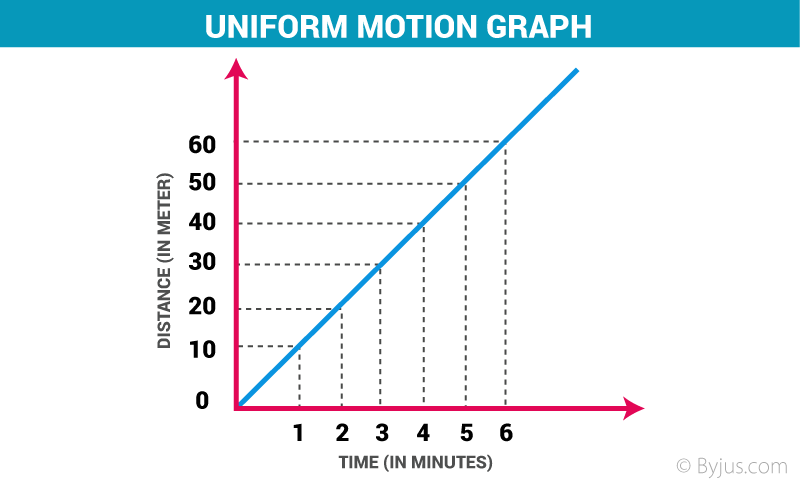Non – Uniform Motion: This type of motion is defined as the motion of an object in which the object travels with varied speed and it does not cover same distance in equal time intervals, irrespective of the time interval duration.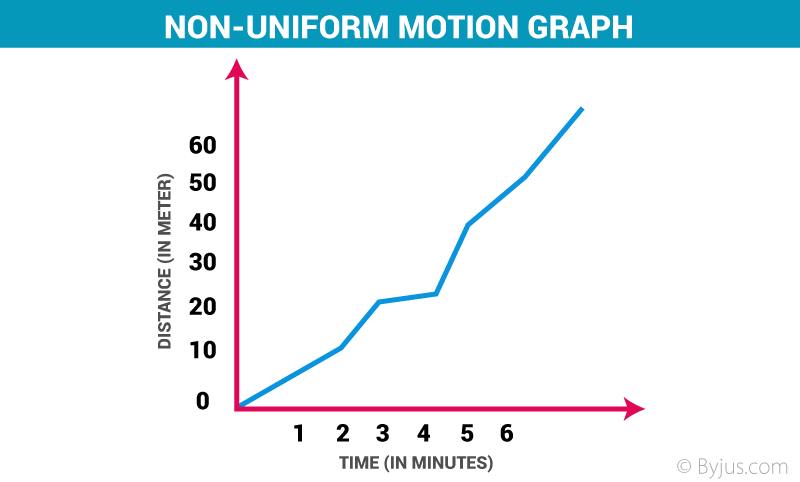The equation of motion with the help of velocity time graph can be accessed from the link provided below:

https://byjus.com/physics/derivation-of-equation-of-motion/

Q54. Define the Equations of Balanced and Unbalanced forces. Explain with the help of

suitable diagram that only unbalanced force can generate motion in an object.

Answer: Balanced Force: A force where two forces of equal size, act on a body, in opposing directions is known as a Balanced Force. In Balanced Force, a body continues to be in its position i.e. it may be continuously moving with the same speed and in the same direction or it may be still in its position.

Example of Balanced Force – Suppose you are pushing a wall and as expected, the wall does not move and neither do you. It means that you and the wall are exerting a balanced force on each other.

To know more about balanced force, students can visit:

https://byjus.com/physics/balanced-force/

Unbalanced Force: A force where two forces acting in opposing directions on a body are not equal in magnitude and in size, is known as an unbalanced force. In an unbalanced force, a body changes its position i.e. we can observe a moving object changing its direction, either increasing the speed or decreasing the speed and a body at rest starts to move and vice versa.

Examples of Unbalanced Force

Practically anything that moves is a result of the exertion of unbalanced forces on it. If you kick a football and it moves from one place to another, it means that unbalanced forces are acting upon it.

Q55. Explain the Newton’s Laws of motion taking examples from day to day life. Establish the relation between force, mass and acceleration on the basis of the Second Law.

Answer: Newton’s First Law of Motion: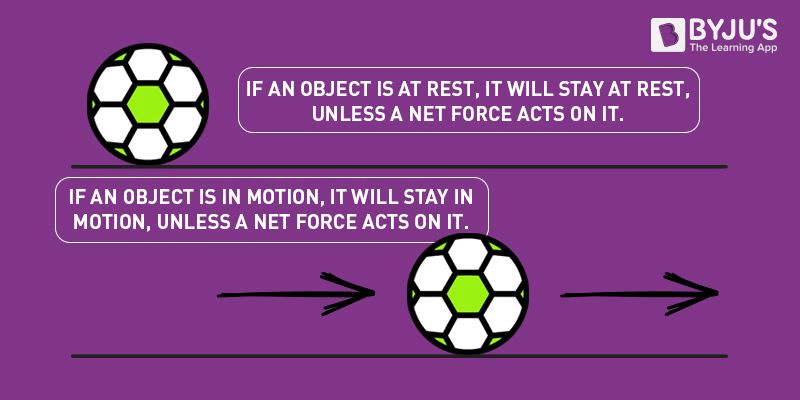Newton’s First Law of Motion: Newton’s first law of motion states that “A body at rest or uniform motion will continue to be at rest or uniform motion until and unless a net external force acts on it”. Suppose a block is kept on the floor, it will remain at rest until we apply some external force to it. Also, we know that it takes us more effort or force to move a heavy mass. This is directly related to a property known as Inertia. This law is also known as the law of inertia.

Example: Wearing a seat belt in a car while driving is an example of Newton’s 1st law of motion. If an accident occurs, or if brakes are applied to the car suddenly, the body will tend to continue its inertia and move forward, probably proving fatal. To prevent such accidents seat belts are used which stops your body moving forward in inertia avoiding danger.

Newton’s Second Law of Motion: According to Newton’s second law of motion, the net force experienced by a body is directly proportional to the rate of change of momentum of the body. It can be written as: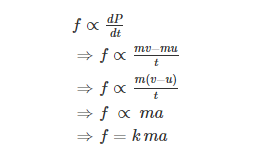Where k is the constant of proportionality and it comes out to be 1 when the values are taken in SI unit. Hence, the final expression will be,

F = ma

Example: Kicking a ball – When we kick a ball we exert force in a specific direction, which is the direction in which it will travel. In addition, the stronger the ball is kicked, the stronger the force we put on it and the further away it will travel.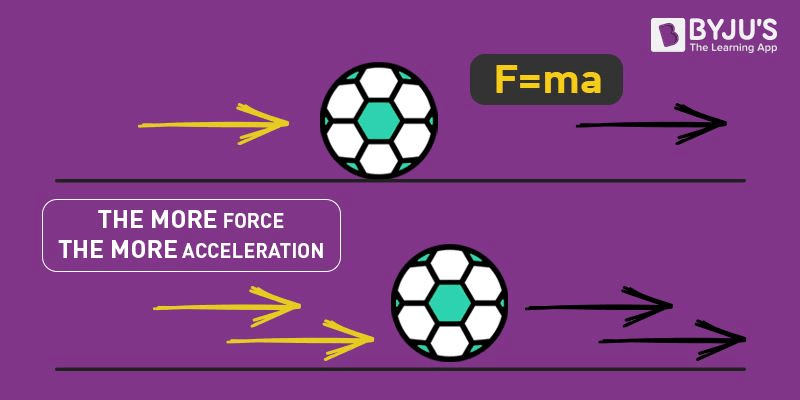Newton’s Third Law of Motion: According to Newton’s third law of motion, for every action, there is an equal and opposite reaction. Forces are always found in pairs. For instance, when you sit on a chair, your body exerts a force downward and that chair needs to exert an equal force upward or else the chair will collapse.

Example: Walking: When you walk, you push the street i.e. you apply a force on the street and the reaction force moves you forward.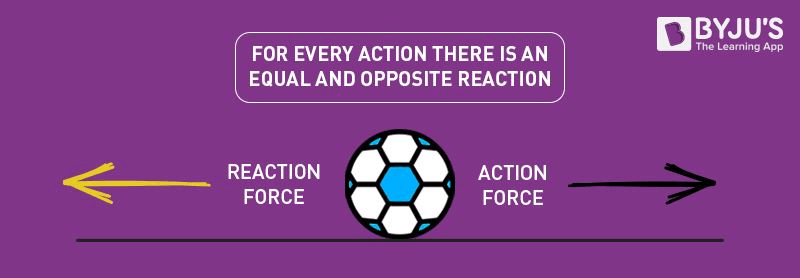Q56. What is meant by Inertia? Explain taking two examples.

Answer: Inertia is an intrinsic characteristic of the object related to its mass. Inertia tells you how much force it will take to cause a particular acceleration on the object. If the body is at rest it will remain at rest and if it is moving, it will continue moving. Inertia are of three types:

• Inertia of Rest
• Inertia of Direction
• Inertia of Motion

Examples:

• The dust particles come out from dirty quilts, mats etc. if they are hung and then struck at with a stick because of this inertia.
• If a horse starts running suddenly the rider falls back. The reason for this also is the inertia of rest.
• The passengers of a bus or a car experience a push towards the back, if the vehicle is suddenly moved from a state of rest. This is because of the inertia of rest of the passenger.

Q57. Define Momentum. With the help of a diagram show that the Momentum is conserved during the direct collision of two moving objects.

Answer: Momentum is how we measure mass that is in motion. Any moving object will have momentum. Under the law of physics, the object’s momentum equals mass times velocity.

Momentum = Mass x Velocity

Momentum is the quantity of motion and is compounded of the quantity of the matter moved, and the velocity with which it moves. Momentum is present when you walk, run, etc. Also, if a bicycle and a car travel down the street at the equal velocity the car will have the most momentum.

Since velocity is considered to be a vector, momentum is considered a vector as well. This means momentum has a significance of momentum and also has a direction. The momentum direction is indicated by a vector or an arrow.

Visit the mentioned link to know more about Conservation of Momentum: https://byjus.com/physics/conservation-of-momentum/

### RBSE Class 9 Science Chapter 9: Numerical Questions and Solutions

Q58. A ship having a mass of 3×107 Kg is in a state of rest. It is pulled to a distance of 3m by applying a force of 5×104 N. If the friction of water is negligible then find the speed of the ship.

Answer: Given, Mass of the hip (m) = 3×107 Kg

Force (F) = 5×104 N

We can calculate acceleration of the ship by using the formula

a = F/m

Therefore, a = 5×104 N / 3×107 Kg

= 5 / 3 ×10-3m/s2

Distance pulled by the ship S = 3m

Initial speed of the ship u = 0

So, using the formula v2 – u2 = 2as

= v2 – 0 = 2 × 5/3 ×10-3 × 3

= v2 = 10-2

⟹ v = 0.1m/sec

Q59. The speed of a bus increases from 25km/h to 70 km/h in 5s. Determine the mean acceleration of the bus.

Time (t) = 5 sec

Initial velocity = 25km/h

Final velocity = 70 km/h

Now, lets convert them in m/s

In first stage

25 × 5/18

= 6.944

In the second stage

70 × 5/18

=19.44

We know that

a = v – u/t

a = 19.44m/s – 6.94m/s

a = 12.5/5 = 2.5m/s2

Q60. The momentum of a child riding a bicycle is 400 kg m/s. The cycle is moving with a velocity of 5 m/s. Find the mass of the child along with the bicycle.

Momentum = 400kg m/s

Velocity = 5 m/s

By using the formula,

Momentum = mass × velocity

Therefore,

400 = mass × 5

mass = 400/5

mass = 80 kg

Q61. A child throws a ball in the upward direction and then catches it after 8 sec. Then determine

(a) the velocity with which the ball was thrown up

(b) At what height will the velocity of the ball will become zero?

(g = 9.8 m/s2)

Answer a: Let’s assume that the initial velocity by which ball was thrown be u.

Then, final velocity v = 0

Acceleration a = 9.8m/s2

From the first equation of motion:

v = u + a × t

v = u + a × t

0 = u + 9.8 × 8

u = 78.4m/s

b) Let’s assume that the height to which ball rises be h.

Then,

v = 0, u = 78.4, a = 9.8 m/s2

From third equation of motion :

v × 2 = u × 2 + 2ah

0 = 78.4 ☓ 78.4 + 2 ☓- 9.8 ☓ h.

0 = 6146.56 – 19.6 h

19.6 h = 6146. 56 m

h = 313.6 m